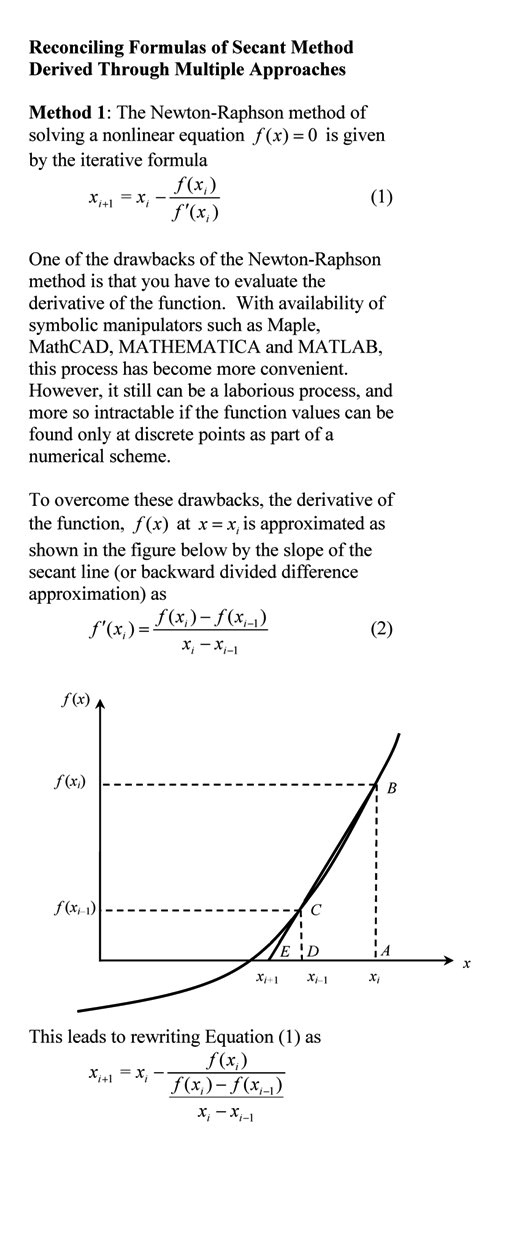## Background Example for Newton Raphson Method

One of the three tenets of a student succeeding in a course is how well he knows the pre-requisite knowledge for the course (other two tenets are ability and interest).  We as instructors can make it easier for students to get to a reasonable competency level by offering short reviews of the pre-requisite knowledge in form of video and/or text.

Here , a student will review via an example the background needed to learn Newton-Raphson method of solving a nonlinear equation of the form f(x)=0.

For more videos and resources on this topic, please visit http://nm.mathforcollege.com/topics/newton_raphson.html

________________________

This post is brought to you by

• Holistic Numerical Methods Open Course Ware:
• the textbooks on
• the Massive Open Online Course (MOOCs) available at

## Reconciling secant method formulas

Depending on how you derive the secant method, one gets the final formulas that are the same but the form is different.  This blog shows one how to reconcile the formulas._______________________________

This post is brought to you by

## A Wolfram demo on how much of a floating ball is under water

Here is another Wolfram demo. This one shows how much of a floating ball would be submerged under water.  The demonstration uses the specific gravity and radius of the ball as inputs.  Using calculus and Archimedes’ principle, the level to which the ball is submerged turns out to be the solution of a cubic equation.  To play with the demo, download the free CDF player from Wolfram first.

This post is brought to you by Holistic Numerical Methods: Numerical Methods for the STEM undergraduate at http://numericalmethods.eng.usf.edu, the textbook on Numerical Methods with Applications available from the lulu storefront, the textbook on Introduction to Programming Concepts Using MATLAB, and the YouTube video lectures available at http://numericalmethods.eng.usf.edu/videos.  Subscribe to the blog via a reader or email to stay updated with this blog. Let the information follow you.

## A real-life example of having to solve a nonlinear equation numerically?_______________________________________________

This post is brought to you by Holistic Numerical Methods: Numerical Methods for the STEM undergraduate at http://numericalmethods.eng.usf.edu, the textbook on Numerical Methods with Applications available from the lulu storefront, and the YouTube video lectures available at http://numericalmethods.eng.usf.edu/videos and http://www.youtube.com/numericalmethodsguy

## How do I solve a nonlinear equation that needs to be setup in MATLAB?

Many students ask me how do I do this or that in MATLAB. So I thought why not have a small series of my next few blogs do that. In this blog, I show you how to solve a nonlinear equation that needs to be set up.

For example to find the depth ‘x’ to which a ball is floating in water is based on the following cubic equation
4*R^3*S=3*x^2*(R-x/3)
where
S= specific gravity of ball
So how do we set this up if S and R are input values?

The MATLAB program link is here.

The HTML version of the MATLAB program is here.

%% HOW DO I DO THAT IN MATLAB SERIES?
% In this series, I am answering questions that students have asked
% me about MATLAB.  Most of the questions relate to a mathematical
% procedure.

%% TOPIC
% How do I solve a nonlinear equation if I need to set it up?

%% SUMMARY

% Language : Matlab 2008a;
% Authors : Autar Kaw;
% Mfile available at
% http://nm.mathforcollege.com/blog/integration.m;
% Last Revised : March 28, 2009;
% Abstract: This program shows you how to solve a nonlinear equation
% that needs to set up as opposed that is just given to you.
clc
clear all

%% INTRODUCTION

disp(‘ABSTRACT’)
disp(‘   This program shows you how to solve’)
disp(‘   a nonlinear equation that needs to be setup’)
disp(‘ ‘)
disp(‘AUTHOR’)
disp(‘   Autar K Kaw of https://autarkaw.wordpress.com&#8217;)
disp(‘ ‘)
disp(‘MFILE SOURCE’)
disp(‘   http://nm.mathforcollege.com/blog/nonlinearequation.m&#8217;)
disp(‘ ‘)
disp(‘LAST REVISED’)
disp(‘   April 17, 2009’)
disp(‘ ‘)

%% INPUTS
% Solve the nonlinear equation where you need to set up the equation
% For example to find the depth ‘x’ to which a ball is floating in water
% is based on the following cubic equation
% 4*R^3*S=3*x^2*(R-x/3)
% S= specific gravity of ball
% So how do we set this up if S and R are input values

S=0.6
R=0.055
%% DISPLAYING INPUTS
disp(‘INPUTS’)
func=[‘  The equation to be solved is 4*R^3*S=3*x^2*(R-x/3)’];
disp(func)
disp(‘  ‘)

%% THE CODE
% Define x as a symbol
syms x
% Setting up the equation
C1=4*R^3*S
C2=3
f=[num2str(C1) ‘-3*x^2*(‘ num2str(R) ‘-x/3)’]
% Finding the solution of the nonlinear equation
soln=solve(f,x);
solnvalue=double(soln);

%% DISPLAYING OUTPUTS

disp(‘OUTPUTS’)
for i=1:1:length(solnvalue)
fprintf(‘\nThe solution# %g is %g’,i,solnvalue(i))
end
disp(‘  ‘)

This post is brought to you by Holistic Numerical Methods: Numerical Methods for the STEM undergraduate at http://nm.mathforcollege.com, the textbook on Numerical Methods with Applications available from the lulu storefront, and the YouTube video lectures available at http://nm.mathforcollege.com/videos